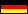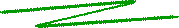The Graph of the Riemann zeta function ζ(s)The Riemann zeta function, in symbols ζ(s), is a rather complicated function which the mathematician Bernhard Riemann (1826-1866) introduced in 1859 to generalize the Euler zeta function

ζ(s) =
 ∞ Σ n=1
n-s         (s > 1)

to the complex plane. Since the Riemann zeta function ζ is a function from the complex plane ℂ to itself, i.e., ζ: ℂ → ℂ, its graph cannot be represented as a 3D image. Instead, the real part and the imaginary part are plotted separately. In addition, the absolute value |ζ(s)| may be shown.

You can download an executable Java program which plots the graph of the ζ function in this way:

(It requires Java JRE 6 or higher).

You can adjust the ranges of the plotted values Re s, Re s, and z, where s = Re s + i Im s, and z = Re ζ(s), z = Im ζ(s), or z = |ζ(s)|, respectively.

In the plot, the real and imaginary lines are projected on the graph and are marked red. The so-called "critical line" (Re s = 1/2) is marked green; the famous Riemann hypothesis conjectures that all zeros besides s = -2, -4, -6, .... lie on the critical line.

If your browser has installed Java Applets, you can quickly start the program by simply clicking the button:

 © de Vries 2004-2015